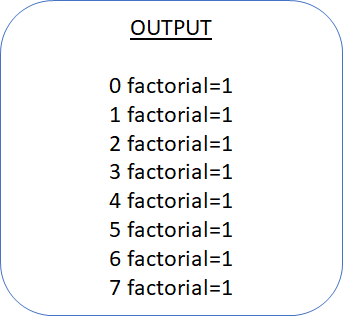# Trung tâm đào tạo thiết kế vi mạch Semicon

• Đăng ký
 Name: * Tên đăng nhập: * Email: * Mật khẩu: * Verify Password: * Fields marked with an asterisk (*) are required.Static: For a variable static lifetime is, its memory never de-allocated until simulation ends. Automatic: For a variable Automatic lifetime is, it is stack storage of variable (for multiple entries to a task, function, or block,

it will have stack storage) and its memory will be de-allocated once execution of that method or block is over.

1. Class variable: Automatic

2. Method variable: Automatic

3. Local variable of the loop: Automatic

4. Module or Program block variable: Static

5. Variable declared in initial or always block: Static

1. Method in module or program block: Static

2. Method in class: Automatic

Let’s understand through the below example.

In below mentioned example factorial function is recursive. But as default it is static it will not create stack entry for it.

module tryfact;

function  integer factorial (input [31:0] operand);

begin

if (operand >= 2)

factorial = factorial (operand - 1) * operand;

else

factorial = 1;

end

endfunction: factorial

integer result;

initial begin

for (int n = 0; n <= 7; n++) begin

result = factorial(n);

\$display("%0d factorial=%0d", n, result);

end

end

endmodule: tryfacta lifetime of method in program block and module is static. This is to keep it the same with the existing Verilog reference. So for module function lifetime is static. Its variable and return type are static. So if we call recursive function, it will not create multiple stack entries for call of function and stack memory for the internal variable.

See in below example where the function is automatic.

module tryfact;

function automatic integer factorial (input [31:0] operand);

begin

if (operand >= 2)

factorial = factorial (operand - 1) * operand;

else

factorial = 1;

end

endfunction: factorial

integer result;

initial begin

for (int n = 0; n <= 7; n++) begin

result = factorial(n);

\$display("%0d factorial=%0d", n, result);

end

end

endmodule: tryfactIn the above example, we explicitly mentioned function as an automatic. So, it will create multiple call entries and variable(related to the method) memory entries. As you can see that in the output of the given example.

Bạn Có Đam Mê Với Vi Mạch hay Nhúng      -     Bạn Muốn Trau Dồi Thêm Kĩ Năng

Mong Muốn Có Thêm Cơ Hội Trong Công Việc

Và Trở Thành Một Người Có Giá Trị Hơn

# Hãy Để Chúng Tôi Hỗ Trợ Cho Bạn. SEMICON

Lần cập nhật cuối ( Thứ bảy, 18 Tháng 9 2021 13:39 )